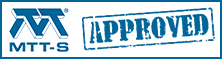# S-parameters

Here's a page on transfer S-parameters, which are more friendly if you want to cascade blocks.

Here's a page covering the most common network parameters and their interrelationships including s-parameters.

Here's a page on mixed-mode S-parameters.

When you come down to it, there are really only a few things that separate a microwave engineer from a "normal" electrical engineer: knowledge of the Smith chart, S-parameters, transmission lines including waveguides, and decibels. Thankfully, these are all simple concepts and we'll help you master them right here at Microwaves101!

### History of S-parameters

We all want to understand and predict how a circuit works, so we want to be able to represent circuit behavior by using mathematical expressions or equations. The starting place is linearity. If a circuit consists of linear elements, then it can be represented by a set of linear equations relating its independent quantities, like currents and voltages, to its dependent quantities, also currents and voltages. This remarkable fact allows us to take a complicated circuit with lots of pieces, put it into a black box, pull out two pairs of nodes defined by voltages and currents, and reduce it all to two linear equations relating these voltages and currents. Before the 1950's and the extensive use of high frequency systems, network parameters like Y- and Z-parameters were the primary method of characterizing circuit performance. At higher frequencies the concept of voltages and currents became more difficult to relate to network performance - especially in networks using transmission lines like waveguides. S-parameters are related to power waves and avoid a lot of these issues. S-parameters refer to the scattering matrix of a microwave network (the "S" in S-parameters refers to scattering). For an early reference, check out the article by E. W. Matthews, Jr working at the Sperry Gyroscope Company called "The Use of Scattering Matrices in Microwave Circuits," where he suggests that the first apparent mention of scattering coefficients was in 1920 in the AIEE article "Maximum Output Networks for Telephone Substation and Repeater Circuits." Click on the link and see if you can find the mention.  For me, the 1948 Radiation Laboratory series, "Principles of Microwave Circuits" introduced the emerging microwave community to practical approaches to solving microwave design problems using scattering parameters in the design of circuits. Click on this link and see if you agree and also see a copy of the entire book courtesy of Google.

It helped that during the 1960s, Hewlett Packard introduced the first microwave network analyzers. The scattering wave concept was further popularized around the time that Kaneyuke Kurokawa of Bell Labs wrote his 1965 IEEE article "Power Waves and the Scattering Matrix."  Also, Robert Collin's textbook Field Theory of Guided Waves, published 1960, has a brief discussion on the Scattering matrix. Collin's book is extensively annotated, including an author index, which reads like a Who's Who of electromagnetic theory for the first half of the twentieth century.

### Introduction to S-parameters

Before we get into the math, let's define a few things you need to know about S-parameters.

The scattering matrix is a mathematical construct that quantifies how RF energy propagates through a multi-port network. The S-matrix is what allows us to accurately describe the properties of incredibly complicated networks as simple "black boxes". For an RF signal incident on one port, some fraction of that signal gets reflected back out of the incident port, some of it enters into the incident port and then exits at (or scatters to) some or all of the other ports (perhaps being amplified or attenuated). What's left of that incident power disappears as heat or even electromagnetic radiation. The S-matrix for an N-port contains N2 coefficients (S-parameters), each one representing a possible input-output path.

S-parameters are complex numbers, having real and imaginary parts or magnitude and phase parts, because both the magnitude and phase of the incident signal are changed by the network. Quite often we refer to the magnitude of the signal only, as it is frequently of most interest. Who cares how the signal phase is changed by an amplifier or attenuator? You mostly care about how much gain (or loss) you get. S-parameters are defined for a given frequency and system impedance, and vary as a function of frequency for any non-ideal network.

S-parameters are usually displayed in a matrix format, with the number of rows and columns equal to the number of ports. For the S-parameter Sij the j subscript stands for the port that is excited (the input port), and the "i" subscript is for the output port. Thus S11 refers to the ratio of the amplitude of the signal that reflects from port one to the amplitude of the signal incident on port one. Parameters along the diagonal of the S-matrix are referred to as reflection coefficients because they only refer to what happens at a single port, while off-diagonal S-parameters are referred to as transmission coefficients, because they refer to what happens at one port when it is excited by a signal incident at another port. Here are the S-matrices for one, two and three-port networks: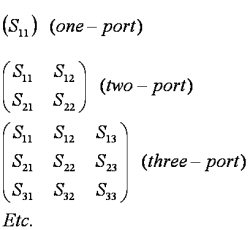Note that each S-parameter is a complex number, so if actual data were presented in matrix format, a magnitude and phase angle would be presented for each Sij.

The input and output reflection coefficients of networks (such as S11 and S22) can be plotted on the Smith chart. Transmission coefficients (S21 and S12) are usually not plotted on the Smith chart.

### Definition of S-parameters

S-parameters describe the response of an N-port network to signal(s) incident to any or all of the ports. The first number in the subscript refers to the responding port, while the second number refers to the incident port. Thus S21 means the response at port 2 due to a signal at port 1. The most common "N-port" networks in microwaves are one-port and two-port networks. Three-port network S-parameters are easy to model with software such as Agilent ADS, but three-port S-parameter measurements are extremely difficult to perform with accuracy. Measured multi-port S-parameters are typically available from vendors for amplifiers and other devices but, as always, make sure you check your answers for reasonableness.

Let's examine a two-port network. The signal at a port, say port 1, can be thought of as the superposition to two waves traveling in opposite directions.  By convention each port is shown as two nodes so as to give a name and value to these opposite direction waves. The variable ai represents a wave incident to port i and the variable bj represent a wave reflected from port j. Don't get all hung up on how two signals can occur at the same node! The magnitude of the ai and bj variables can be thought of as voltage-like variables, normalized using a specified reference impedance.  This is very convenient since the square of these magnitudes are then equal to the power level of the waves. Remember, S-parameters don't mean much unless you know the value of the reference impedance (it's frequently called Z0).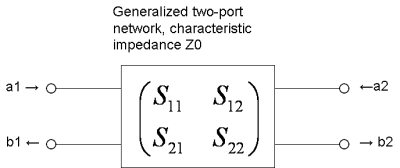If we assume that each port is terminated in the reference impedance Z0, we can define the four S-parameters of the 2-port as: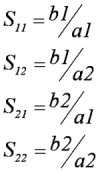The above equations for S11 and S21 are derived from network analysis or measurements by setting the value of the incident signal a2=0 and solving for the above S-parameter ratios as a function of a1. Similarly, S12 and S22 are derived by setting the value of a1=0 and solving for the other ratios.

See how the subscript neatly follows the parameters in the ratio (S11=b1/a1, etc...)? Here's the matrix algebraic representation of 2-port S-parameters: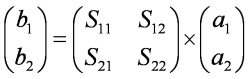If we want to measure S11, we inject a signal at port one and measure its reflected signal. In this case, no signal is injected into port 2, so a2=0; for almost all laboratory S-parameter measurements, we only inject one signal at a time. If we want to measure S21, we inject a signal at port 1, and measure the resulting signal power exiting port 2. For S12 we inject a signal into port 2, and measure the signal power leaving port 1, and for S22 we inject a signal at port 2 and measure its reflected signal.

Did we mention that all the a and b measurements are complex numbers? It isn't always necessary to keep track of the angle of the S-parameters, but complex S-parameters are a much more powerful tool than magnitude-only S-parameters, and the math is simple enough either way. By the way, these complex numbers are sometimes called vectors, hence the term vector network analyzers (VNA).

S-parameter magnitudes are presented in one of two ways, linear magnitude or logarithmic based decibels (dB). Because S-parameters are complex voltage ratios, the formula for decibels in this case is

Sij(dB)=20 * log[Sij(magnitude)]

Remember that power ratios are expressed as 10 * log(whatever). Voltage ratios are 20 * log(whatever), because power is proportional to voltage squared.

The angle or phase of a complex S-parameter is almost always presented in degrees (but, of course, radians are possible).

### Types of S-parameters

When we are talking about networks that can be described with S-parameters, we are usually talking about single-frequency networks. Receivers and mixers aren't referred to as having S-parameters, although you can certainly measure the reflection coefficients at each port and refer to these parameters as S-parameters. The trouble comes when you wish to describe the frequency-conversion properties, this is not possible using S-parameters.

Small signal S-parameters are what we are talking about 99% of the time. By small signal, we mean that the signals have only linear effects on the network, small enough so that gain compression or other non-linear effects do not take place. For passive networks, small-signal is all you have to worry about, because they act linearly at any power level (at least until you blow them up).

Large signal S-parameters are more complicated. In this case, the S-matrix will vary depending upon the input signal strength. Measuring and modeling large signal S-parameters will not be described on this page (perhaps we will get into that someday)

Mixed-mode S-parameters refer to a special case of analyzing balanced circuits. We're not going to get into that either!

Pulsed S-parameters are measured on power devices so that an accurate representation is captured before the device heats up. This is a tricky measurement, and not something we're gonna tackle yet.

### Cold S-parameters

Information on the  S-parameters of cold or unpowered networks starts on this page.

### Other matrices

S-parameters are just one of many matrix representations of a network that can fully describe it. Besides S-parameters, networks can be described by matrix representations which include ABCD parameters, Y-parameters and Z-parameters. ABCD parameters are frequently used "behind the scenes" in many calculations, because they are easily cascadable. By cascadable, we mean that if you want to simulate an attenuator followed by an amplifier, the S-parameter math will drive you insane, while the ABCD math involves nothing more than multiplication. This will remain a topic for another day!

Come back soon!

Author : Unknown Editor